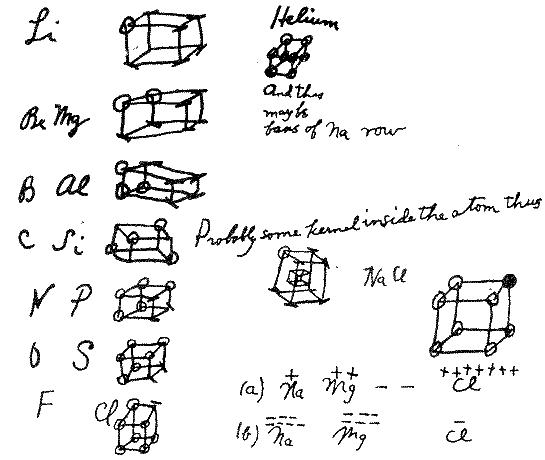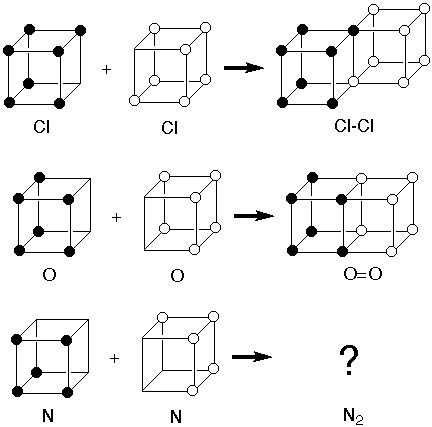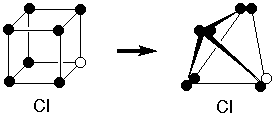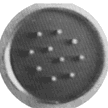Two Things Wrong with Lewis's Cubic Octet StructuresG.N.Lewis proposed the cubic octet in 1902 as a chemistry teaching tool to give a structural "explanation" for the periodicity of the elements in terms of the electron, which physicist J.J. Thomson had discovered only seven years previously. Here are his notes from 1902.

As we will see again and again in the course, when someone proposes a model to explain one set of phenomena, the next step is to see if it can be used to understand other phenomena. Lewis tried to stretch his model for periodicity and ionic charge to rationalize the number of covalent bonds that an atom can form - its valence.

But there were two problems:

1). The model was consistent with formation of the Cl-Cl single bond to complete the octet of two chlorine atoms by sharing two electrons along an edge. It was also consisent with formation of the O=O double bond by sharing four electrons across a face. But there was no way it was consistent with formation of a triple bond in N2.Lewis nimbly got around this obstruction by supposing that instead of having individual electron sites at the corners of a cube, there were pairs of electron sites at the corners of a tetrahedron. So two tetrahedra could share three corners to form a triple bond. This also seemed consistent with the previously known tetrahedral geometry of the tetravalent carbon.2) There is an even more fundamental objection to the very idea of any static structure for the atom.

In 1839 Samuel Earnshaw had proven that no system governed by inverse square force laws (i.e. where energy is proportional to 1/r and force, the derivative of energy to 1/r2) can possess the local energy minimum that would be necessary if a particle is to have a stable location. Many important forces are of the 1/r2 form. These include gravity, the interaction between charges, and the interaction between magnetic poles.

(Click for What are Force Laws? )

Lewis, like leading physicists J.J. Thomson and James Jeans, speculated that over very small distances, such as those within an atom, the energy of interacting charges might no longer obey Coulomb's Law (E = q1q2/r12), and that this would allow escape from the Earnshaw restriction. In 1923 Thomson wrote:

"… if [electron-nuclear attraction] were to vary strictly as the inverse square of the distance we know by Earnshaw's theorem than no stable configuration in which the electrons are at rest or oscillating about positions of equilibrium is possible ..."

 "I shall assume that the law of force between a positive charge and an electron is expressed by the equation"… then a number of electrons can be in equilibrium about a positive charge without necessarily describing orbits around it."

J. J. Thomson,The Electron in Chemistry (1923)

(Notice that Thomson arbitrarily multiplies Coulomb's Force Law by a factor that is essentially unity for large distance r, but changes the sign of the force when r becomes smaller than c, a constant which he assumed was about the radius of an atom. This would have been brilliant, if it had only been correct.)

In the same year the chemist Lewis wrote:

"...if we use the electron as a test charge to determine the properties of the simplest possible electric field, namely the field about a hydrogen nucleus, we appear to find that this field is not a continuum but is strikingly discontinuous."

"Instead of thinking then of an electric field as a continuum, we should rather regard it as an intensely complicated mesh."

G.N.Lewis,Valence and the Structure of Atoms and Molecules (1923)

But Lewis, Thomson, and Jeans were wrong. Coulomb's Law does apply down to very small distances (<10-20 times the size of an atom). A set of point charges cannot have a stable static structure, cubic octet or whatever, in the absence of non-1/r2 forces.

Of course the particles in an atom are not static. What turned out to be wrong with classical physics as applied to such small systems was its formulation of kinetic energy as 1/2 mv2. This was corrected just three years later in 1926 by Quantum Mechanics, as we'll soon see when we address the true structure of the atom.

Another Model of Shell Structure

Like Lewis, J. J. Thomson was intrigued by periodicity and its possible interpretation in terms of a shell structure for electrons. Like other British physicists he loved physical models of fundamental phenomena. He would have appreciated Blonder's model of the shell structure for charged balls.

 Click here to see Blonder's 2-D model in which electrically charged balls that repel one another but are confined within a fixed area (through repulsion by a surrounding charged ring) form patterns that show shell structure. Blonder's apparatus is described here.[In case you're interested, the following links show how two toys achieve levitation
by circumventing Earnshaw's (correct) Theorem: Levitron ; Diamagnetic Levitator ]